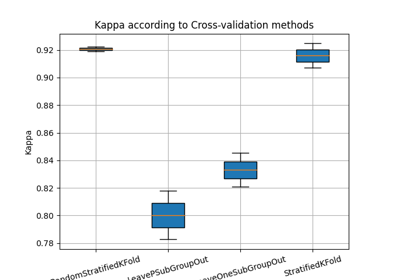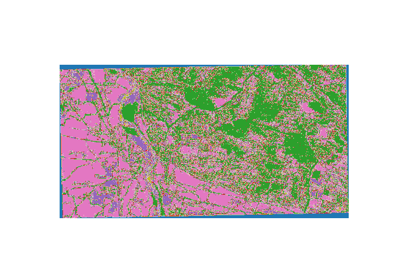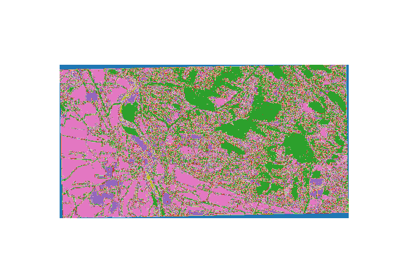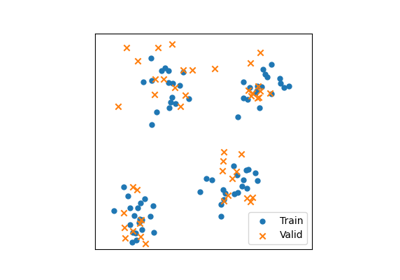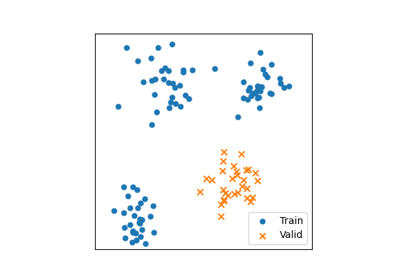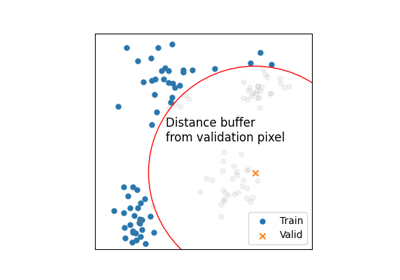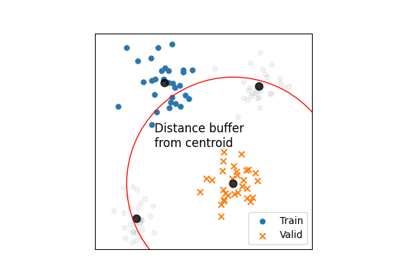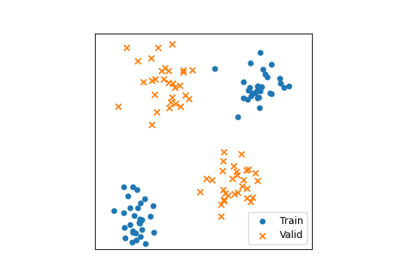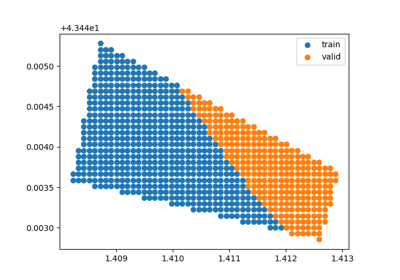# extract_ROI¶

`museotoolbox.processing.``extract_ROI`(in_image, in_vector, *fields, **kwargs)[source]

Extract raster values from Regions Of Interest in a vector file. Initially written by Mathieu Fauvel, improved by Nicolas Karasiak.

Parameters
• in_image (str.) – the name or path of the raster file, could be any file that GDAL can open.

• in_vector (str.) – A filename or path corresponding to a vector file. It could be any file that GDAL/OGR can open.

• *fields (str.) – Each field to extract label/value from.

• **kwargs (list of kwargs.) –

• get_pixel_positionbool, optional (default=False).

If get_pixel_position=True, will return pixel position in the image for each point.

• only_pixel_positionbool, optional (default=False).

If only_pixel_position=True, with only return pixel position for each point.

• prefer_memorybool, optional (default=False).

If prefer_memory=False, will write temporary raster on disk to extract ROI values.

• verbosebool or int, optional (default=True).

The higher is the int verbose, the more it will returns informations.

Returns

• X (np.ndarray, size of (n_samples,n_features).) – The sample matrix. A n*d matrix, where n is the number of referenced pixels and d is the number of features. Each line of the matrix is a pixel.

• y (np.ndarray, size of (n_samples,).) – The label of each pixel.

`museotoolbox.processing.read_vector_values()`

read field values from vector file.

Examples

```>>> from museotoolbox.datasets import load_historical_data
>>> from museotoolbox.processing import extract_ROI
>>> X,Y = extract_ROI(raster,vector,'Class')
>>> X
array([[ 213.,  189.,  151.],
[ 223.,  198.,  158.],
[ 212.,  188.,  150.],
...,
[ 144.,  140.,  105.],
[  95.,   92.,   57.],
[ 141.,  137.,  102.]])
>>> X.shape
(12647,3)
>>> Y
[3 3 3 ..., 1 1 1]
>>> Y.shape
(12647,)
```

## Examples using `museotoolbox.processing.extract_ROI`¶